# How to Measure Capacity Versus Bias Voltage on MLCCs

### Abstract

The application note explains how to always check the capacitor’s datasheet to see how the capacitance varies with the bias voltage. Using the presented circuit, a dual power supply, and a voltmeter, it is quite simple to measure the DC bias characteristic of a high-capacity MLCC.

### Introduction

High-capacity, multilayer ceramic capacitors (MLCC) have a property often not well understood by electronic designers: the capacitance of these devices varies with applied DC voltage. This phenomenon is present in all high-dielectric constant, or Class II capacitors (B/X5R R/X7R, and F/Y5V characteristic). However, the amount of variation can differ considerably among different MLCC types. A good application note on this topic was written by Mark Fortunato.

The conclusion of this application note is that you should always check the capacitor's datasheet to see how the capacitance varies with the bias voltage. But what if the data sheet does not include this information? How can you determine how much capacitance is lost under the conditions in your application?

### Theory for Characterizing Capacitance versus Bias Voltage

A circuit to measure the DC bias characteristic is shown in Figure 1.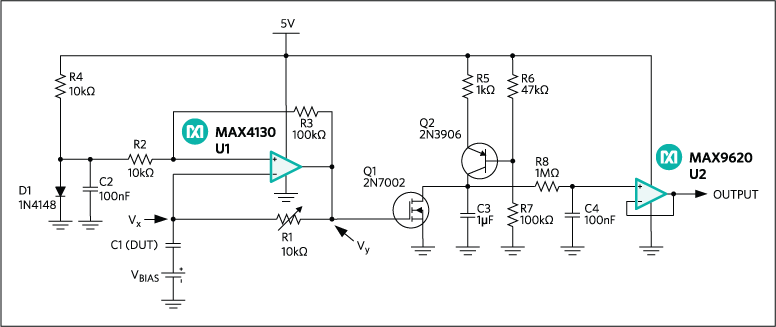This circuit is built around op amp, U1 (MAX4130). The op amp acts as a comparator with feedback resistors R2 and R3 adding hysteresis. D1 sets a threshold above GND so that no negative supply voltage is needed. C1 and R1 form a feedback network to the negative input, which makes the circuit operate as an RC oscillator. Capacitor C1, the device under test (DUT), serves as the C in this RC oscillator; potentiometer R1 is the R.

The voltage waveforms of the op amp output pin, Vy, and the junction between R and C, Vx, are shown in Figure 2. When the output of the op amp is at 5V, capacitor C1 is charged by R1 until it reaches the upper threshold; thus, forces the output to 0V. Now the capacitor is discharged until Vx reaches the lower threshold, thus forcing the output back to 5V. This process repeats, resulting in a stable oscillation.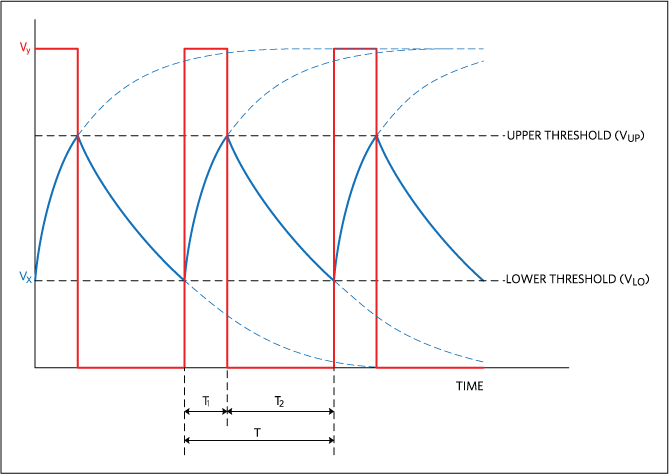The oscillation period depends on the values of R, C, and the upper and lower thresholds VUP and VLO: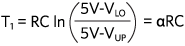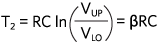Since 5V, VUP, and VLO are constant, then T1 and T2 are proportional to RC. This is often referred to as the RC time constant.

The threshold of the comparator is a function of Vy, R2, R3, and the forward voltage of D1 (VDIODE):where VUP is the threshold for Vy = 5V, and VLO is the threshold for Vy = 0V. With the given values these thresholds yield to approximately 0.55V for VLO, and 1.00V for VUP.

The circuit around Q1 and Q2 converts the cycle time into a proportional voltage. This works as follows. MOSFET Q1 is controlled by the output of U1. During T1, Q1 is on, clamping the voltage on C3 to GND. During T2, Q1 is off, allowing the constant current source (Q2, R5, R6, and R7) to linearly charge C3.1 As T2 is increased, the voltage on C3 becomes higher. Figure 3 shows the voltage on C3 over three cycles.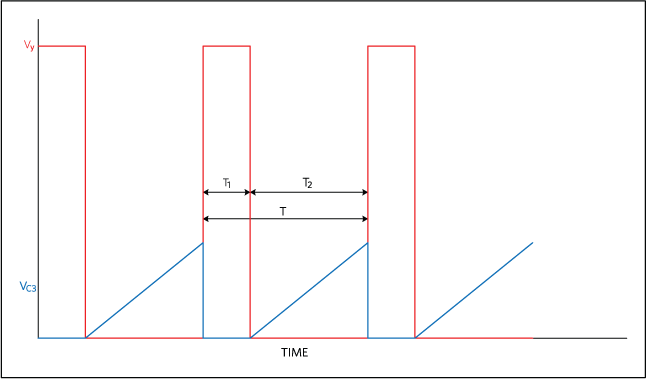The average voltage on C3 (VC3) is equal to:Since I, C3, α , and β are all constant, the average voltage on C3 is proportional to T2 and, therefore, also to C1.

Lowpass filter R8/C4 filters the signal while low-offset op amp U2 (MAX9620) buffers the output so that it can be measured with any voltmeter.

Before measurements can be made, this circuit requires a simple calibration. First the DUT is installed in the circuit, and VBIAS is set to 0.78V (the average of VLO and VUP) so the actual average (DC) voltage across the DUT is 0V. The output voltage will vary when potentiometer R1 is varied. Adjust R1 until the output voltage reads 1.00V. Under these conditions, the peak voltage on C3 is around 2.35V.2 The bias voltage can be modified and the output voltage will show the resultant percentage change in the capacitance. For example, if the output voltage is 0.80V, the capacitance at that particular bias voltage is 80% of the capacitance at 0V bias.

#### Lab Tests Confirm the Theory

The Figure 1 circuit was built on a small PCB. The first measurement was done using a random 10µF capacitor. Figure 4 and Figure 5 show the signals under 0V and 5V bias conditions, respectively.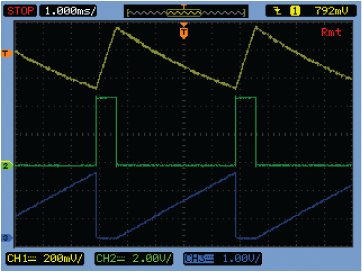To prevent saturation of Q2, the voltage peak on the collector (= VC3) should stay below the emitter voltage minus the emitter-collector saturation voltage, which yields to approximately 4V.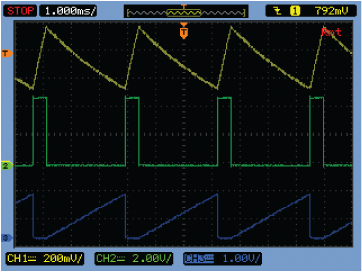At 0V bias, potentiometer R1 was adjusted so the voltmeter showed 1.000V. At 5V bias, the voltmeter showed 0.671V, indicating that 67.1% of the capacitance remained. With an accurate counter, the total period, T, was also measured. T was 4933µs at 0V bias and 3278µs at 5V, indicating that 66.5% (= 3278µs/4933µs) of the capacitance remained. These values match very well, demonstrating that the circuit design can accurately measure the capacitance drop as a function of the bias voltage.

A second measurement was performed, now using a known 2.2µF/16V capacitor taken from a sample kit supplied by Murata (part number = GRM188R61C225KE15). In this measurement the values were recorded over the entire operating 0 to 16V range. The relative capacitance was determined by measuring both the output voltage of the circuit and the actual oscillation period. Additionally, data was collected from the Murata® Simsurfing tool, which can provide the DC bias characteristic for this particular part based on measurements performed by Murata. Figure 6 shows all the results. Both graphs with our measurement data show almost identical results, which proves that the time-to-voltage circuit performs well over a larger dynamic range. There is some difference between the data from the Simsurfing tool and our measurements, but the shapes of the curves are similar.### Conclusion

Using the presented circuit, a dual power supply, and a voltmeter it is quite simple to measure the DC bias characteristic of a high-capacity MLCC. A quick bench test will reveal how much the capacitance decreases as a result of the applied bias voltage.

### Footnotes

1. This will only be linear when using a capacitor with constant capacitance up to 5V bias voltage (MKS, MKT, etc).
2. To prevent saturation of Q2, the voltage peak on the collector (= VC3) should stay below the emitter voltage minus the emitter-collector saturation voltage, which yields to approximately 4V.

### References

Fortunato, Mark, "Temperature and Voltage Variation of Ceramic Capacitors," EDN, December 4, 2012, http://www.techonline.com/electrical-engineers/education-training/tech-papers/4410874/Temperature-and-Voltage-Variation-of-Ceramic-Capacitors. Also found as Maxim Integrated application note 5527, "Temperature and Voltage Variation of Ceramic Capacitors, or Why Your 4.7µF Capacitor Becomes a 0.33µF Capacitor," by Mark Fortunato.